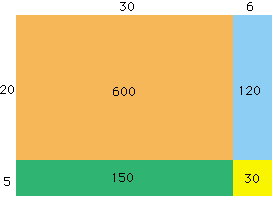Sender: John Ost Hello, I am a college student taking an elementary math course I need if possible assistance to developing an area model 36 x 25 that shows each of the four separate partial products. I must know how to do the computation 36x25 showing each of the four partial products separately, and indicate how each corresponds to the drawing that is required of the area model. This is for grades K-5 any help is appreciated. Sincerely, John Ost Hi John, If what you want is to think of 36x25 as (30 + 6)x(25 + 5) = 600 + 150 + 120 + 30 then the diagram below is a nice way to view the four partial products.Cheers, Penny Go to Math Central